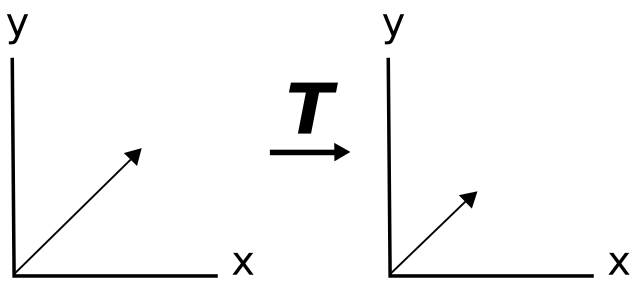# Eigenvector

## What is an Eigenvector?

Eigen, meaning ‘characteristic of’ or ‘peculiar to’, describes a set of values, vectors, spaces and functions,  that fulfill the same related definition. Here we consider eigenvectors which fulfill the following definition. A transformation t (which operates on and creates vectors) has a scalar eigen value  if there is a vector (not zero)  such that t()= . Intuitively this can be understand as a system where the only thing that happens to  is simple multiplication by . This is important because the identity of  is still preserved and can be recovered by dividing out .

## Finding Eigenvalues and Vectors of a Matrix T

Limiting ourselves to the assumption t is a matrix T. We find the Eigenvalues and Eigenvectors of the system T= by first finding all Eigenvalues which make the determinant of the matrix [T-I] and solving for 0. Once we have these values we can find the solution of  (T-I)=0for each  which will yield generic forms for the eigenvectors corresponding to that eigenvalue. Which is to say that for a given eigenvalue , any of its corresponding Eigenvectors will be equal to when transformed by T.

The graphic below shows a vector which is an eigenvector of the transformation T. Note that the vector does not change its location but only its length.### Eigenvalues and Vectors in Machine Learning

In machine learning, it is important to choose features which represent large amounts data points and give lots of information. Picking the features which represent that data and eliminating less useful features is an example of dimensionality reduction. We can use eigenvalues and vectors to identify those dimensions which are most useful and prioritize our computational resources toward them.